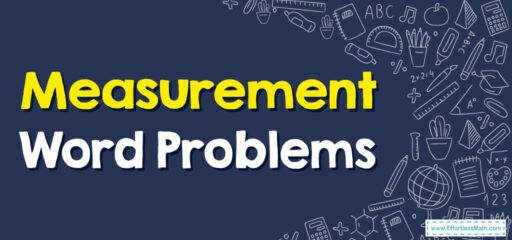# How to Solve Measurement Word Problems

Measurement word problems often involve understanding and using different units of measurement. These problems might ask you to convert from one unit to another or to find a total, difference, or product of given measurements.## A Step-by-step Guide to Solving Measurement Word Problems

Here’s a simple step-by-step guide for a student to solve measurement word problems:

### Step 1: Understand the Problem

First, read the problem carefully. Try to understand what the problem is asking you to do. Look for keywords or phrases that indicate what operation you should use (like ‘total’ for addition or ‘difference’ for subtraction).

### Step 2: Identify the Units and Numbers

Next, write down all the measurements given in the problem, including their units (like meters, grams, hours, etc.). Also, note any numbers that might be important.

### Step 3: Decide on the Operation(s)

Determine what operation or operations you need to perform to find the answer. It could be addition, subtraction, multiplication, or division. In some cases, you might need to convert one unit to another.

### Step 4: Perform the Operation(s)

Do the math! If you need to convert units, do that first. Then perform the necessary operation or operations.

Finally, write down your answer, making sure to include the correct unit. Make sure your answer makes sense in the context of the problem.

### What people say about "How to Solve Measurement Word Problems - Effortless Math: We Help Students Learn to LOVE Mathematics"?

No one replied yet.

X
30% OFF

Limited time only!

Save Over 30%

SAVE $5 It was$16.99 now it is \$11.99